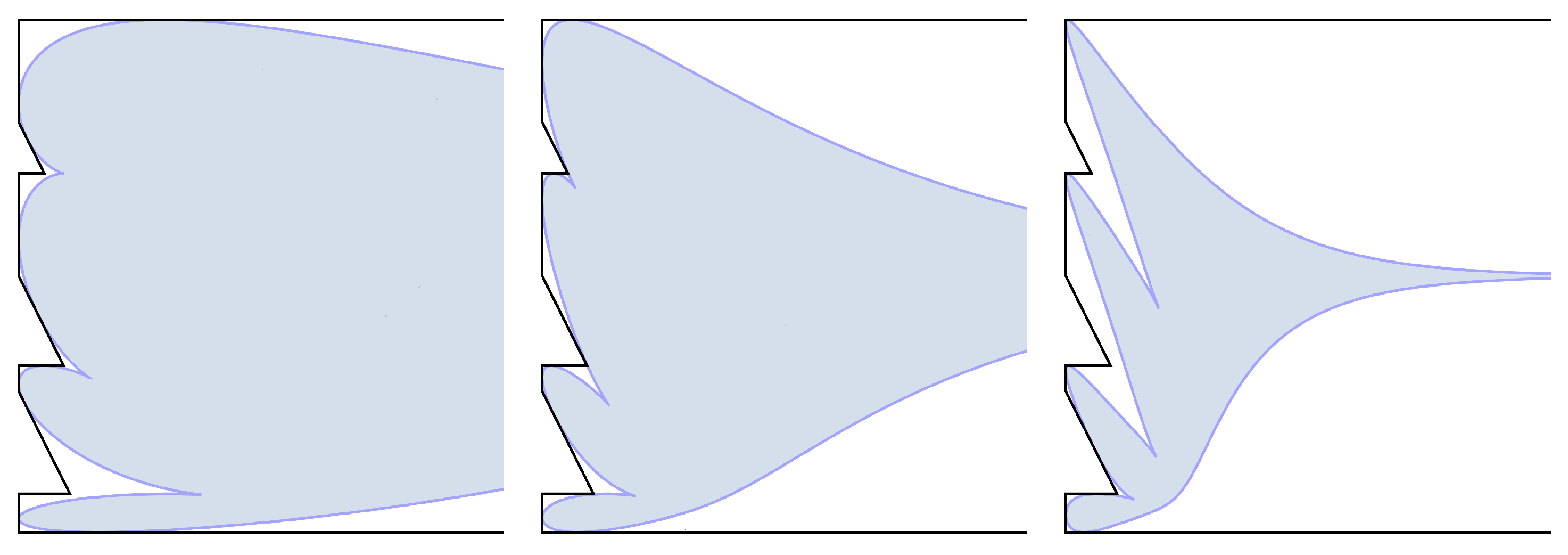# Asymptotics of noncolliding q-exchangeable random walks

### 2023/03/03

Leonid Petrov, Mikhail Tikhonov
J. Phys. A: Math. Theor. 56 365203arXiv:2303.02380 [math.PR]

PDF

We consider a process of noncolliding $q$-exchangeable random walks on $\mathbb{Z}$ making steps $0$ (straight) and $-1$ (down). A single random walk is called $q$-exchangeable if under an elementary transposition of the neighboring steps $(\textnormal{down},\textnormal{straight}) \to (\textnormal{straight}, \textnormal{down})$ the probability of the trajectory is multiplied by a parameter $q\in(0,1)$. Our process of $m$ noncolliding $q$-exchangeable random walks is obtained from the independent $q$-exchangeable walks via the Doob’s $h$-transform for a certain nonnegative eigenfunction $h$ with the eigenvalue less than $1$. The system of $m$ walks evolves in the presence of an absorbing wall at $0$.

We show that the trajectory of the noncolliding $q$-exchangeable walks started from an arbitrary initial configuration forms a determinantal point process, and express its kernel in a double contour integral form. This kernel is obtained as a limit from the correlation kernel of $q$-distributed random lozenge tilings of sawtooth polygons.

In the limit as $m\to \infty$, $q=e^{-\gamma/m}$ with $\gamma>0$ fixed, and under a suitable scaling of the initial data, we obtain a limit shape of our noncolliding walks and also show that their local statistics are governed by the incomplete beta kernel. The latter is a distinguished translation invariant ergodic extension of the two-dimensional discrete sine kernel.Limit shapes of the noncolliding q-exchangeable random walks